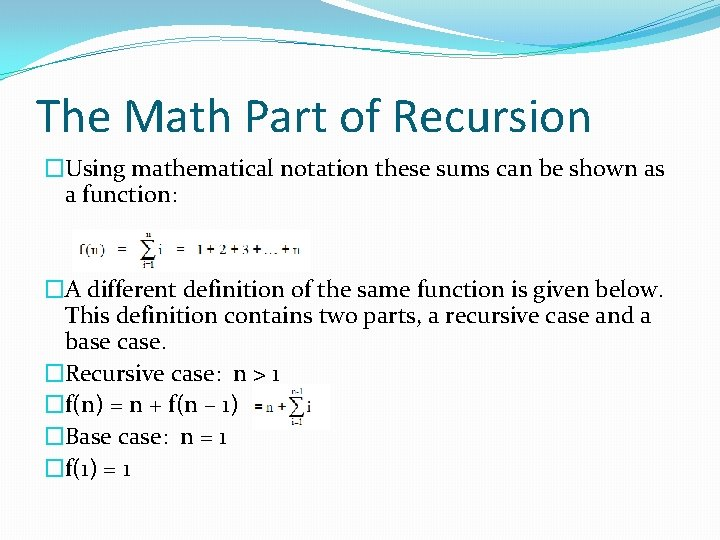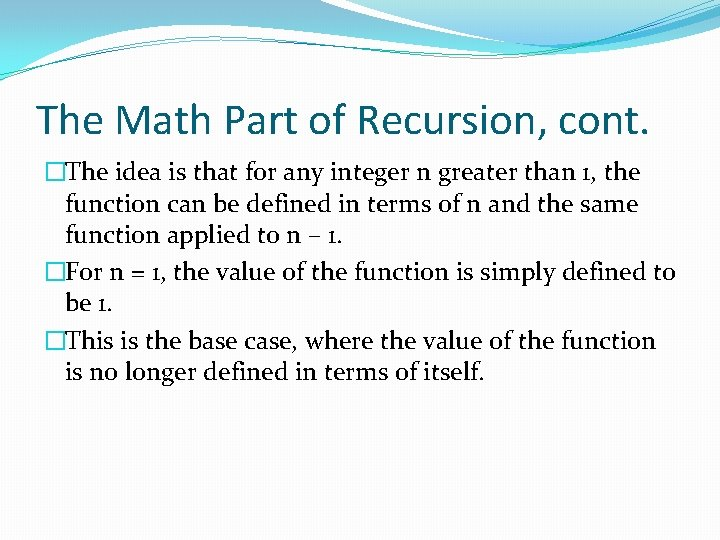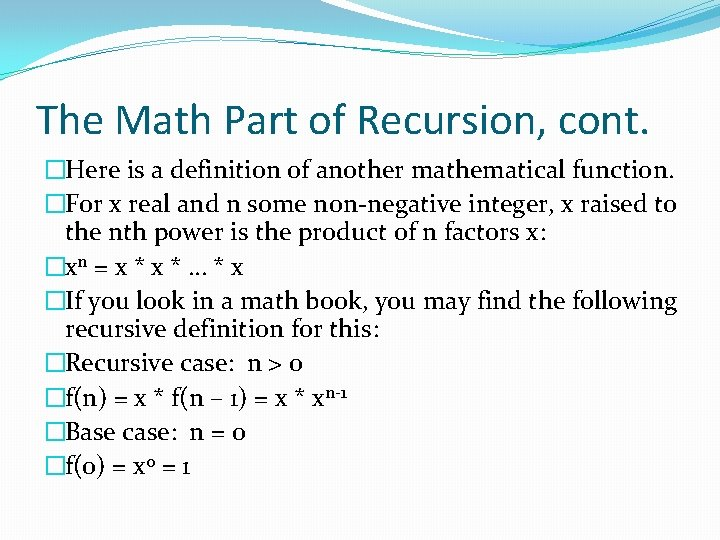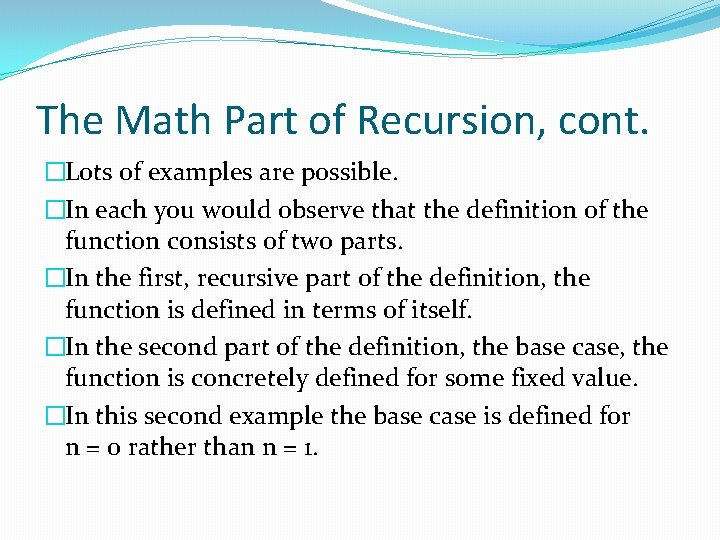# 10 1 Description of Recursion Recursion When a

• Slides: 1010. 1 Description of RecursionRecursion � When a method contains a call to itself, this is referred to as recursion. � Consider the following class containing the skeleton of a static method: �public class My. Class �{ � public static int my. Method(int parameter. One) � { � int parameter. Two; � … � return my. Method(parameter. Two); � } �}Recursion, cont. �my. Method() contains a call to itself. �Notice that syntactically, it’s not necessary to write the call as My. Class. mymethod(…) because the call occurs within code belonging to My. Class. �Recursion can be defined simply as a method that calls itself.Rack of Pool Balls Example �The picture shown below represents a rack of pool balls. It will be used to illustrate how a recursive definition can be developed for the summation of positive integers. � � �Rack of Pool Balls Example, cont. �You can ask the following question as you move from the top row down: How does the total number of balls in the rack depend on the number of rows? �For a rack containing 5 rows, it’s easy enough to figure this out and put the information in a little table: � # of rows � 1 � 2 � 3 � 4 � 5 sum of balls in rows 1 1+2+3+4+5 total number of balls in rack 1 3 6 10 15 � � �The Math Part of Recursion �Using mathematical notation these sums can be shown as a function: �A different definition of the same function is given below. This definition contains two parts, a recursive case and a base case. �Recursive case: n > 1 �f(n) = n + f(n – 1) �Base case: n = 1 �f(1) = 1The Math Part of Recursion, cont. �The idea is that for any integer n greater than 1, the function can be defined in terms of n and the same function applied to n – 1. �For n = 1, the value of the function is simply defined to be 1. �This is the base case, where the value of the function is no longer defined in terms of itself.The Math Part of Recursion, cont. �Here is a definition of another mathematical function. �For x real and n some non-negative integer, x raised to the nth power is the product of n factors x: �xn = x * … * x �If you look in a math book, you may find the following recursive definition for this: �Recursive case: n > 0 �f(n) = x * f(n – 1) = x * xn-1 �Base case: n = 0 �f(0) = x 0 = 1The Math Part of Recursion, cont. �Lots of examples are possible. �In each you would observe that the definition of the function consists of two parts. �In the first, recursive part of the definition, the function is defined in terms of itself. �In the second part of the definition, the base case, the function is concretely defined for some fixed value. �In this second example the base case is defined for n = 0 rather than n = 1.The Math Part of Recursion, cont. �In the examples above, n was a positive integer. �In the recursive part of the definition, f(n) was defined in terms of n and the f(n – 1). �In other words, f(n) was defined in terms of an application of the function to a value 1 less. �The base case defined f(n) for n = 0 or n = 1. �These examples illustrate the general structure of recursion. �n is decreased by 1 in each successive application of the recursive function. �It is critical that n reach the value of the base case so that the recursion will stop.